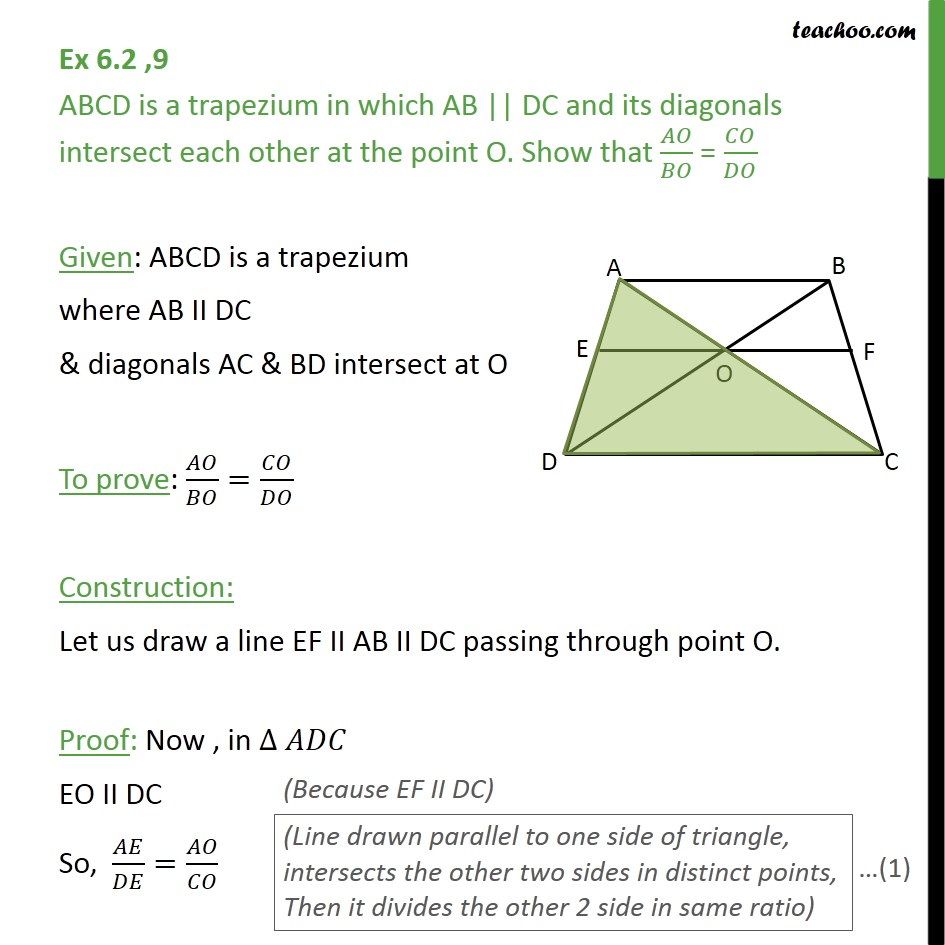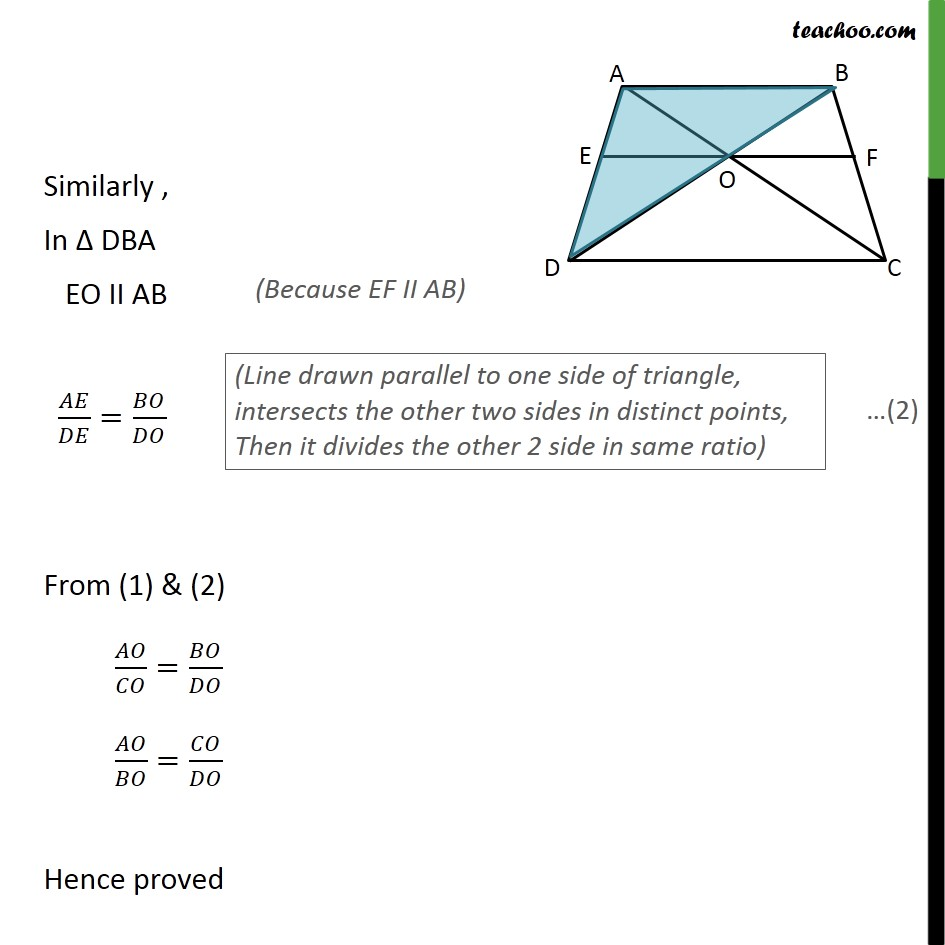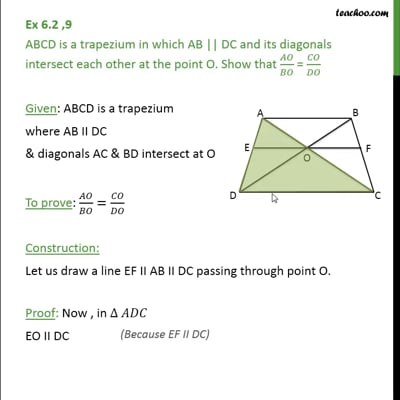Ex 6.2

Chapter 6 Class 10 Triangles (Term 1)
Serial order wiseThis video is only available for Teachoo black users

### Transcript

Ex 6.2 ,9 ABCD is a trapezium in which AB || DC and its diagonals intersect each other at the point O. Show that / = / Given: ABCD is a trapezium where AB II DC & diagonals AC & BD intersect at O To prove: / = / Construction: Let us draw a line EF II AB II DC passing through point O. Proof: Now , in EO II DC So, / = / Similarly , In DBA EO II AB / = / From (1) & (2) / = / / = / Hence proved